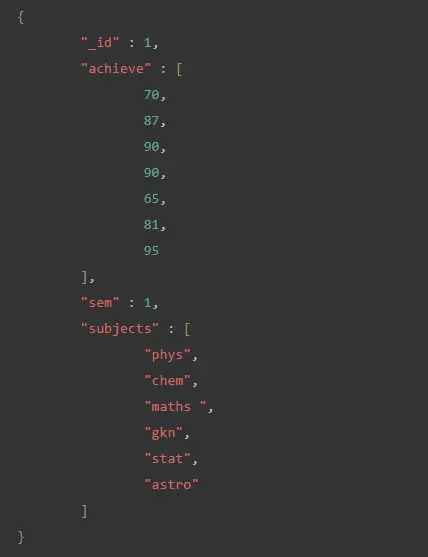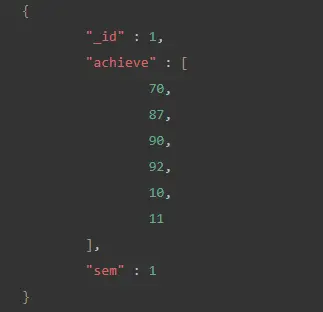# Difference Between \$push and \$addToSet in MongoDB

Tahseen Tauseef Apr 28, 2022

This article explains what operators are in MongoDB. What does `\$push` and `\$addToSet` operators are used for.

Additionally, the difference between these two operators is given with code segments. This article discusses the following topics.

1. Operators in MongoDB
2. `\$push` in MongoDB
3. `\$addToSet` in MongoDB

## Operators in MongoDB

Operators are special symbols or keywords that inform a compiler or an interpreter to carry out mathematical or logical operations.

The query operators enhance the functionality of MongoDB by allowing developers to create complex queries to interact with data sets that match their applications.

Different types of operators in MongoDB are explained below.

1. Query and Projection Operators - Query operators aid in discovering data in a database, whereas projection operators alter how data is shown.
2. Update Operators - Update operators allow you to change or add data to your database.
3. Aggregation Pipeline Stages - Available aggregation stages for Aggregation Pipeline.
4. Aggregation Pipeline Operators - Aggregation pipeline operations have a collection of operators available to define and manipulate documents in pipeline stages.
5. Query Modifiers - Query modifiers determine how queries will be executed.

## `\$push` Operator in MongoDB

The `\$push` operator in MongoDB is used to attach a given value to an array. A type of update operator is the `\$push` operator.

If the designated field is not present in the document to be updated, the `\$push` operator adds it as a new field with the specified value as its element. The operation failed if the updating field was not of the array type.

If the value is an array, the `\$push` operator appends the entire array as a single element when updating. If you wish to add each value element independently, use the `\$push` operator with the `\$each` modifier.

Syntax:

``````db.collection.update( <query>,{ \$push: { <field>: <value> } })
``````

The following database collection will be used in all the examples.

Sample Collection:

``````db={
"student": [
{
"_id": 1,
"sem": 1,
"subjects": [
"phys",
"chem",
"maths",
"gkn",
"stat",
"astro"
],
"achieve": [
70,
87,
90,
90,
65,
81
]
}
]
}
``````

### Example of MongoDB `\$push` Operator

If you want to append 95 to the array field accomplished when the condition `subjects` is `"maths"`, we may use the MongoDB command below.

``````db.student.update( { "subjects" : "maths" },{ \$push: { "achieve": 95 } });
``````

Because the criterion described in the preceding example matches with this action, the value 95 will be appended to the array accomplished.

To see the newly updated document:

``````db.student.find().pretty();
``````

The output of the query given above can be seen in this screenshot.### Example of MongoDB `\$push` When the Field Is Not an Array

If you wish to add 2 to the `sem` column, which is not an array type field, we may use the MongoDB command below.

``````db.student.update( { "subjects" : "maths" },{ \$push: { "sem": 2 } });
``````

Because the `sem` field in the above example is not of the array type, the operation will fail and produce the following result.

``````Cannot apply \$push/\$push All modifier to non-array
``````

### Example of MongoDB `\$push` Using `\$each` Modifiers

If we want to append many entries or more than one element (`77,49,83`) to the array accomplished for the documented student where the condition `subjects` is `"maths"`, we may use the MongoDB command below.

``````db.student.update( { "subjects" : "maths" },{ \$push: { "achieve": {\$each : [77,49,83 ]} } });
``````

The `\$each` modifier was used in the above example to append several entries `77,49,83` to the array accomplish that matches the criterion topic `"maths"`.

To see the newly updated document:

``````db.student.find().pretty();
``````

The output of the query given above can be seen in this screenshot.## `\$addToSet` in MongoDB

The `\$addToSet` operator only adds or appends a value to an array if the value does not already exist in the array. When the value is already in the array, `\$addToSet` returns the same array without altering it.

The `\$addToSet` operator assures no duplicate entries in an array when it is updated; however, the order of the elements in a display can be modified after adding a value.

Syntax:

``````db.collection.update( { <field>: <value> }, { \$addToSet: { <field>: <addition> } } );
``````

Collection used above:

`````` { "_id" : 1, "sem" : 1, "achieve" : [  80,  70,  90 ] }
``````

### Example of `\$addToSet` to Append a Value

If we want to append the value 92 to the array, we may use the MongoDB command below.

``````db.student.update( { "sem": 1}, { \$addToSet: { "achieve": 92 } } );
``````

The preceding code will append 92 to the array field accomplish.

To see the newly updated document:

``````db.student.find().pretty();
``````

Output of the command:

``````{ "_id" : 1, "achieve" : [ 70, 80, 90, 92 ], "sem" : 1 }
``````

### Example of `\$addToSet` to Add Multiple Value Using `\$each` Modifier

The following MongoDB command may append the numbers 10 and 11 to the array field accomplish.

``````db.student.update( { "sem": 1}, { \$addToSet: { "achieve":{\$each:[10,11]}}});
``````

In the above example, the numbers 10 and 11 were attached to the array field achieved using the `\$each` modifier.

To see the newly updated document:

``````db.student.find().pretty();
``````

The output of the query given above can be seen in this screenshot.